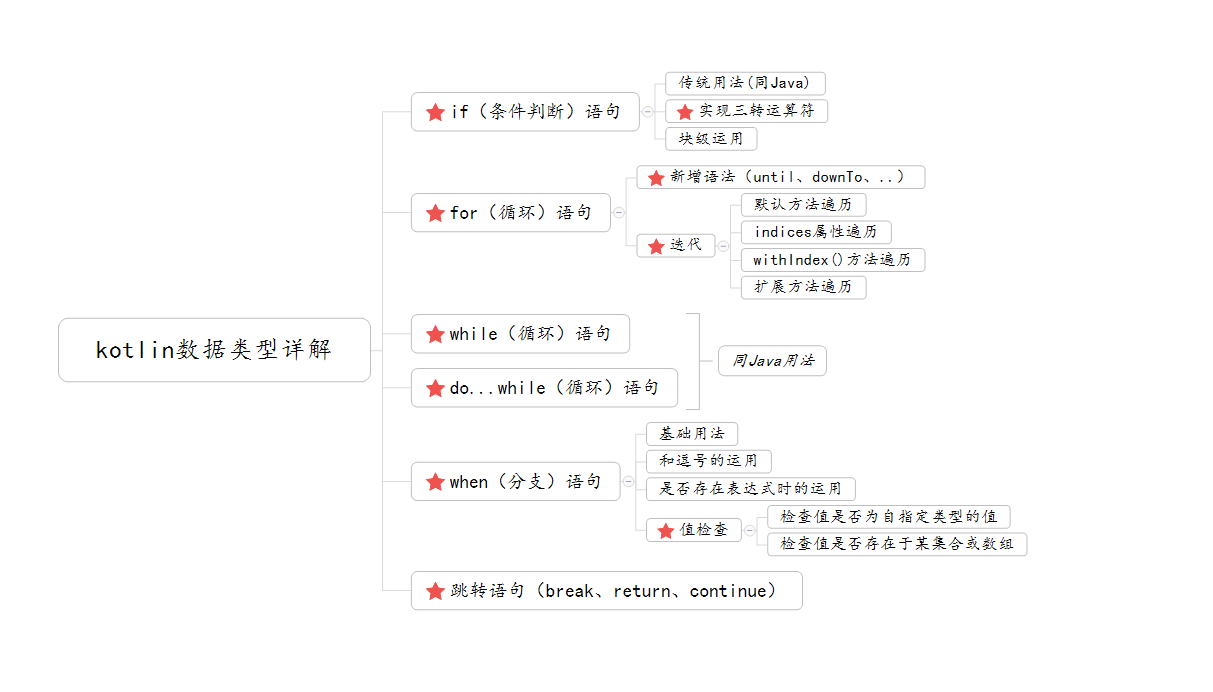# Kotlin——初级篇（四）：控制语句详解## 目录## 一、if语句

Kotlin中的if语句和Java还是还是有一定的区别的，它能在Java中更灵活，除了能实现Java写法外，还可以实现表达式（实现三元运算符），及作为一个块的运用。

1、传统写法（同Java写法一样）

var numA = 2
if (numA == 2){
println("numA == $numA => true") }else{ println("numA ==$numA => false")
}


numA == 2 => true


2、Kotlin中的三元运算符

• 在Kotlin中其实是不存在三元运算符(condition ? then : else)这种操作的。
• 那是因为if语句的特性(if表达式会返回一个值)故而不需要三元运算符。

// 在Java中可以这么写，但是Kotlin中直接会报错。
// var numB: Int = (numA > 2) ? 3 : 5

// kotlin中直接用if..else替代。例：
var numB: Int = if ( numA > 2 ) 3 else 5  // 当numA大于2时输出numB的值为3，反之为5
println("numB = > $numB")  输出结果为： numB = > 3  由上可以看出，Kotlin中的if可以作为一个表达式并返回一个值。 3、作为一个块结构，并且最后一句表达式为块的值 例： var numA: Int = 2 var numC: Int = if (numA > 2){ numA++ numA = 10 println("numA > 2 => true") numA }else if (numA == 2){ numA++ numA = 20 println("numA == 2 => true") numA }else{ numA++ numA = 30 println("numA < 2 => true") numA } // 根据上面的代码可以看出，每一个if分支里面都是一个代码块，并且返回了一个值。根据条件numC的值应该为20 println("numC =>$numC")


numA == 2 => true
numC => 20


## 二、for语句

• Kotlin废除了Java中的for(初始值;条件；增减步长)这个规则。但是Kotlin中对于for循环语句新增了其他的规则，来满足刚提到的规则。
• for循环提供迭代器用来遍历任何东西
• for循环数组被编译为一个基于索引的循环，它不会创建一个迭代器对象

1、新增的规则，去满足for(初始值;条件;增减步长)这个规则

• 1.1、递增

  // 循环5次，且步长为1的递增
for (i in 0 until 5){
print("i => $i \t") }  输出结果为 i => 0 i => 1 i => 2 i => 3 i => 4  • 1.2、递减 • 关键字：downTo • 范围：downTo[n,m] => 即小于等于n,大于等于m ,n > m 例： // 循环5次，且步长为1的递减 for (i in 15 downTo 11){ print("i =>$i \t")
}


i => 15 	i => 14 	i => 13 	i => 12 	i => 11


1.3、符号（' .. ' ） 表示递增的循环的另外一种操作

• 使用符号( '..').
• 范围：..[n,m]=> 即大于等于n，小于等于m
• until的区别，一是简便性。二是范围的不同。

print("使用 符号..的打印结果\n")
for (i in 20 .. 25){
print("i => $i \t") } println() print("使用until的打印结果\n") for (i in 20 until 25){ print("i =>$i \t")
}


使用 符号..的打印结果
i => 20 	i => 21 	i => 22 	i => 23 	i => 24 	i => 25

i => 20 	i => 21 	i => 22 	i => 23 	i => 24


1.4、设置步长

for (i in 10 until 16 step 2){
print("i => $i \t") }  输出结果为： i => 10 i => 12 i => 14  2、迭代 • for循环提供一个迭代器用来遍历任何东西。 • for循环数组被编译为一个基于索引的循环，它不会创建一个迭代器对象 2.1、遍历字符串 此用法在数据类型章节中的字符串类型中用到过。还不甚清楚的可以查看Kotlin——初级篇（三）：数据类型详解 例： for (i in "abcdefg"){ print("i =>$i \t")
}


i => a 	i => b 	i => c 	i => d 	i => e 	i => f 	i => g


2.2、遍历数组

var arrayListOne = arrayOf(10,20,30,40,50)
for (i in arrayListOne){
print("i => $i \t") }  输出结果为： i => 10 i => 20 i => 30 i => 40 i => 50  2.3、使用数组的indices属性遍历 例： var arrayListTwo = arrayOf(1,3,5,7,9) for (i in arrayListTwo.indices){ println("arrayListTwo[$i] => " + arrayListTwo[i])
}


arrayListTwo => 1
arrayListTwo => 3
arrayListTwo => 5
arrayListTwo => 7
arrayListTwo => 9


2.4、使用数组的withIndex()方法遍历

var arrayListTwo = arrayOf(1,3,5,7,9)
for ((index,value) in arrayListTwo.withIndex()){
println("index => $index \t value =>$value")
}


index => 0 	 value => 1
index => 1 	 value => 3
index => 2 	 value => 5
index => 3 	 value => 7
index => 4 	 value => 9


2.5、使用列表或数组的扩展函数遍历

• 数组或列表有一个成员或扩展函数iterator()实现了Iterator<T>接口，且该接口提供了next()hasNext()两个成员或扩展函数
• 其一般和while循环一起使用
1. 可以查看Array.kt这个类。可以看见其中的iterator()函数，而这个函数实现了Iterator接口。

 /**
*   Creates an iterator for iterating over the elements of the array.
*/
public operator fun iterator(): Iterator<T>

2. 查看Iterator.kt这个接口类，这个接口提供了hasNext()函数和next()函数。

 public interface Iterator<out T> {

/**
* Returns the next element in the iteration.
*/
public operator fun next(): T

/**
* Returns true if the iteration has more elements.
*/
public operator fun hasNext(): Boolean
}


var arrayListThree = arrayOf(2,'a',3,false,9)
var iterator: Iterator<Any> = arrayListThree.iterator()

while (iterator.hasNext()){
println(iterator.next())
}


2
a
3
false
9


## 三、when语句

• Kotlin中已经废除掉了Java中的switch语句。而新增了when(exp){}语句。
• when语句不仅可以替代掉switch语句，而且比switch语句更加强大

3.1、when语句实现switch语句功能

when(5){
1 -> {
println("1")
}
2 -> println("2")
3 -> println("3")
5 -> {
println("5")
}
else -> {
println("0")
}
}


5


3.2、和逗号结合使用，相当于switch语句中的不使用break跳转语句

when(1){
// 即x = 1,2,3时都输出1。
1 , 2 , 3 -> {
println("1")
}
5 -> {
println("5")
}
else -> {
println("0")
}
}


1


3.3、条件可以使用任意表达式，不仅局限于常量

var num:Int = 5
when(num > 5){
true -> {
println("num > 5")
}
false ->{
println("num < 5")
}
else -> {
println("num = 5")
}
}


num < 5


3.4、 检查值是否存在于集合或数组中

• 操作符：
1. （in）
2. (!in) 不在
• 限定:只适用于数值类型

var arrayList = arrayOf(1,2,3,4,5)
when(1){
in arrayList.toIntArray() -> {
println("1 存在于 arrayList数组中")
}
in 0 .. 10 -> println("1 属于于 0~10 中")
!in 5 .. 10 -> println("1 不属于 5~10 中")
else -> {
println("都错了 哈哈！")
}
}


元素1存在于 arrayList数组中


3.5、检查值是否为指定类型的值

• 操作符
1. （is）
2. 不是（!is）
• 值得注意的是，Kotlin的智能转换可以访问类型的方法和属性

when("abc"){
is String -> println("abc是一个字符串")
else -> {
println("abc不是一个字符串")
}
}

// 智能转换
var a: Int = 2
when(a){
!is Int -> {
println("$a 不是一个Int类型的值") } else -> { a = a.shl(2) println("a =>$a")
}
}


abc是一个字符串
a => 8


3.6、不使用表达式的when语句

var array = arrayOfNulls<String>(3)
when{
true -> {
for (i in array){
println("循环了$count 次") count++ num++ }  输出结果为： num => 5 循环了1 次 num => 6 循环了2 次 num => 7 循环了3 次 num => 8 循环了4 次 num => 9 循环了5 次  ## 五、do...while语句 • 其同Java中的do...while循环一样。在此不做累述。 • 定义格式： do(exp){ // 其中exp为表达式 ... }(while)  例： var num = 5 var count = 1 do { println("num =>$num")
println("循环了$count 次") count++ num++ }while (num < 10)  输出结果为： num => 5 循环了1 次 num => 6 循环了2 次 num => 7 循环了3 次 num => 8 循环了4 次 num => 9 循环了5 次  PS: do{...}while(exp)与while(exp){...}最大的区别是do{...}while(exp)最少执行一次，这点也是和Java相同的 例： var num = 5 var count = 1 do { println("num =>$num")
println("循环了$count 次") count++ num++ }while (num < 5)  输出结果为： num => 5 循环了1 次  ## 六、跳转语句（return、break、continue） 其同Java中的跳转语句一样。在此不做累述。 1、return语句 默认情况下，从最近的封闭函数或匿名函数返回。 例： fun returnExample(){ var str: String = "" if (str.isBlank()){ println("我退出了该方法") return } }  输出结果为： 我退出了该方法  2、break语句 作用：终止最近的闭合循环。 例： var count: Int = 1 for (i in 1 until 10){ if (i == 5){ println("我在第$i 次退出了循环")
break
}
count++
}
println("我循环了多少次：count => $count")  输出结果为： 我在第5 次退出了循环 我循环了多少次：count => 5  3、continue语句 前进到最近的封闭循环的下一个步骤(迭代)。 例：  for (i in 1 until 10){ if (i == 5){ println("我跳过了第$i 次循环")
continue
}
println("i => \$i")
}


i => 1
i => 2
i => 3
i => 4

i => 6
i => 7
i => 8
i => 9


GithubJteictors

## 欢迎各位大佬进群共同研究、探索

#### QQ群号：497071402posted @ 2017-10-24 09:40  Jetictors  阅读(10566)  评论(2编辑  收藏  举报